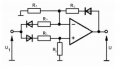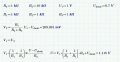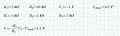# OP amp - double absolute value

#### gmazur

Joined Oct 15, 2019
2
HelloI'm having problems with finding a way to change the circuit attached to make it's output double the value of the input. In this moment I know that for:
R1=R2=R3=R4=R5=R ->U=|U1|
And I need to change resistors value to attain:
U=2|U1|Thanks in advance for any advice#### crutschow

Joined Mar 14, 2008
32,932
That's a rather poor rectifier circuit.
Was that given to you as homework?

Since this is homework please show us your attempt at a solution.
We do Homework Help here, not Homework Do.

Hint: You can solve it with two simultaneous equations: one for the gain when the input is positive, and one for the gain when the input is negative.

Last edited:

#### gmazur

Joined Oct 15, 2019
2
Yes, it is a homeworkTo be honest I'm having a problem even with the equation for the positive input, I've tried to solve it like that:Assuming V1 as inverting input and V2 as non-inverting input.
But I'm very much unsure about how to account for the diodes, I'm quite sure that without the diodes it would be correct.
And in case of negative voltage I've assumed that there is no influence from the non-inverting input:Negative part seems to be correct, but I'm very much in doubt about the positive part.
Voltage drop on the diode is of course just an example value.

#### MrAl

Joined Jun 17, 2014
10,617
Yes, it is a homeworkTo be honest I'm having a problem even with the equation for the positive input, I've tried to solve it like that:
View attachment 232767
Assuming V1 as inverting input and V2 as non-inverting input.
But I'm very much unsure about how to account for the diodes, I'm quite sure that without the diodes it would be correct.
And in case of negative voltage I've assumed that there is no influence from the non-inverting input:
View attachment 232768
Negative part seems to be correct, but I'm very much in doubt about the positive part.
Voltage drop on the diode is of course just an example value.
Do you know how to solve for the gain of a non inverting amplifier?
Also, do you know how to analyze a circuit with a voltage controlled voltage source in it?
Either of these should help just add the effects of the diodes.